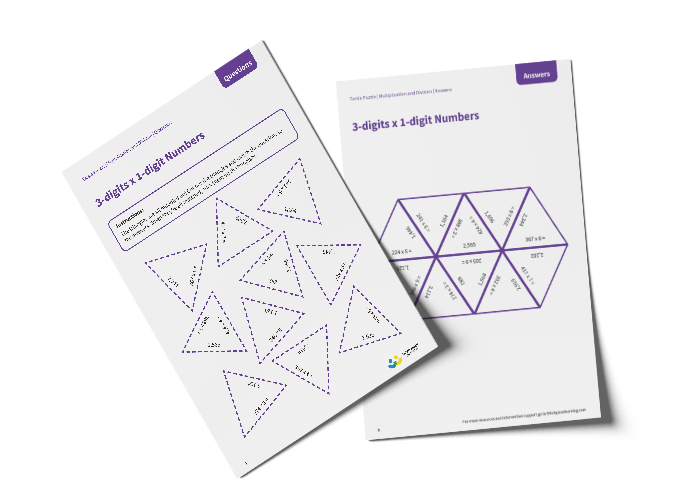# Tarsia Puzzle 3-digit Multiplied by 1-digit Numbers (Year 4)

The triangles in the tarsia puzzle have all been mixed up. In this 3-digit multiplied by 1-digit numbers tarsia puzzle, pupils will need to match the multiplication question to the correct answer to create one large hexagon.

This Year 4, 3-digit multiplied by 1-digit numbers tarsia puzzle worksheet contains:

• One question sheet
• One template sheet

Year 4

Multiplication